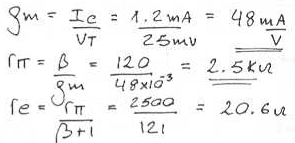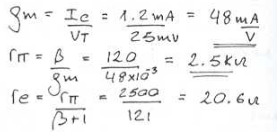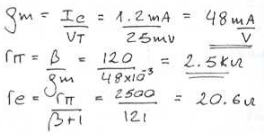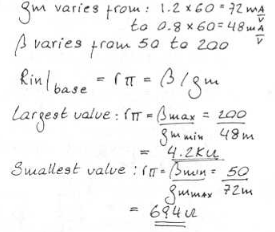Courses

# Test: Small Signal Operations & Model

## 10 Questions MCQ Test Electronic Devices | Test: Small Signal Operations & Model

Description
This mock test of Test: Small Signal Operations & Model for Electrical Engineering (EE) helps you for every Electrical Engineering (EE) entrance exam. This contains 10 Multiple Choice Questions for Electrical Engineering (EE) Test: Small Signal Operations & Model (mcq) to study with solutions a complete question bank. The solved questions answers in this Test: Small Signal Operations & Model quiz give you a good mix of easy questions and tough questions. Electrical Engineering (EE) students definitely take this Test: Small Signal Operations & Model exercise for a better result in the exam. You can find other Test: Small Signal Operations & Model extra questions, long questions & short questions for Electrical Engineering (EE) on EduRev as well by searching above.
QUESTION: 1

### A transistor with ß = 120 is biased to operate at a dc collector current of 1.2 mA. Find the value of gm.

Solution:QUESTION: 2

### A transistor with ß = 120 is biased to operate at a dc collector current of 1.2 mA. Find the value of R?p.

Solution:QUESTION: 3

### A transistor with ß = 120 is biased to operate at a dc collector current of 1.2 mA. Find the value of Re.

Solution:QUESTION: 4

A transistor operating with nominal gm of 60 mA/V has a ß that ranges from 50 to 200. Also, the bias circuit, being less than ideal, allows a 20% variation in Ic. What is the smallest value found of the resistance looking into the base?

Solution:QUESTION: 5

A transistor operating with nominal gm of 60 mA/V has a ß that ranges from 50 to 200. Also, the bias circuit, being less than ideal, allows a 20% variation in Ic. What is the largest value found of the resistance looking into the base?

Solution:
QUESTION: 6

A designer wishes to create a BJT amplifier with a gm of 50 mA/V and a base input resistance of 2000 O or more. What is the minimum ß he can tolerate for the transistor used?

Solution:
QUESTION: 7

A designer wishes to create a BJT amplifier with a gm of 50 mA/V and a base input resistance of 2000 O or more. What emitter bis current should he choose?

Solution:
QUESTION: 8

Which of the following is true?

Solution:

The correct relationship between Ic and Ie is Ib = Ic/ß.

QUESTION: 9

The SI units of transconductance is

Solution:

Transcoductance is given by Ic/Vt.

QUESTION: 10

Which of the following represents the correct mathematical form of the term denoted by the symbol Rp?

Solution:

Both of the expressions are identical.